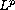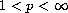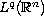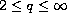Electron. J. Diff. Eqns., Vol. 2006(2006), No. 48, pp. 1-16.

###-resolvent estimates and time decay for generalized thermoelastic plate equations Robert Denk, Reinhard Racke

Abstract:
We consider the Cauchy problem for a coupled system generalizing the thermoelastic plate equations. First we prove resolvent estimates for the stationary operator and conclude the analyticity of the associated semigroup in-spaces,, for certain values of the parameters of the system; here the Newton polygon method is used. Then we prove decay rates of the-norms of solutions,, as time tends to infinity.

Submitted September 13, 2005. Published April 11, 2006.
Math Subject Classifications: 35M20, 35B40, 35Q72, 47D06, 74F05.
Key Words: Analytic semigroup in; polynomial decay rates; Cauchy problem.

Show me the PDF file (306K), TEX file, and other files for this article.Robert Denk Department of Mathematics and Statistics University of Konstanz, 78457 Konstanz, Germany email: robert.denk@uni-konstanz.de Reinhard Racke Department of Mathematics and Statistics University of Konstanz, 78457 Konstanz, Germany email: reinhard.racke@uni-konstanz.de